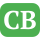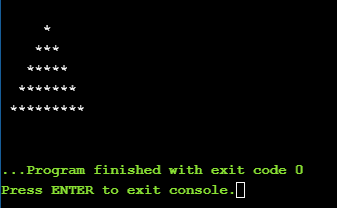CppBuzz.com

# C++ code to print triangle using for loop

This program uses three for loops to print Triangle of *. Program can be done in other loops like while & do-while loops

``````#include<iostream>
using namespace std;

int main()
{
for(int i=5; i>0; i--)
{
for(int j=0; j<=(5-i)*2+1; j++)
{
cout<<"*";
}
cout<<endl;
}
return 0;
}
``````

## Printing Triangle of * in C++